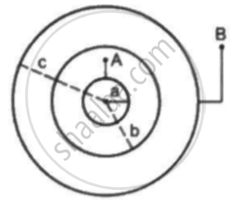Department of Pre-University Education, Karnataka course PUC Karnataka Science Class 12
Share

# Suppose the Space Between the Two Inner Shells of the Previous Problem is Filled with a Dielectric of Dielectric Constant K. Find the Capacitance of the System Between a and B. - Physics

ConceptCapacitors and Capacitance

#### Question

Suppose the space between the two inner shells is filled with a dielectric of dielectric constant K. Find the capacitance of the system between A and B.#### Solution

Since the space between the two inner shells is filled with a dielectric, capacitance CAB  becomes C_(AB) = (4pi∈_0abK)/((b-a)) and capacitance CBC becomes C_(BC) = (4pi∈_0bc)/((c-b)) . Now, as the capacitors are in series, the equivalent capacitance is given by

1/C = 1/C_(AB) + 1/C_(BC)

⇒ C = (C_(AB)C_(BC))/(C_(AB)+C_(BC))

⇒ C = (((4pi∈_0)^2 ab^2 Kc)/((b-a)(c-b)))/((4pi∈_0abk)/((b-a))+(4pi∈_0bc)/((c-d)))

⇒ C = ((4pi∈_0Kab^2c)/((b-c)(c-b)))/(((abk(c-b)+bc(b-a))/((b-a)(c-b))))

⇒ C = (4pi∈_0Kab^2c)/[[abk(c-b)+bc(b-a)]]

⇒ C = (4pi∈_0Kabc)/[[ak(c-b)+c(b-a)]]

Is there an error in this question or solution?

#### Video TutorialsVIEW ALL 

Solution Suppose the Space Between the Two Inner Shells of the Previous Problem is Filled with a Dielectric of Dielectric Constant K. Find the Capacitance of the System Between a and B. Concept: Capacitors and Capacitance.
S